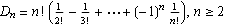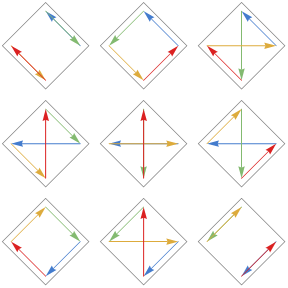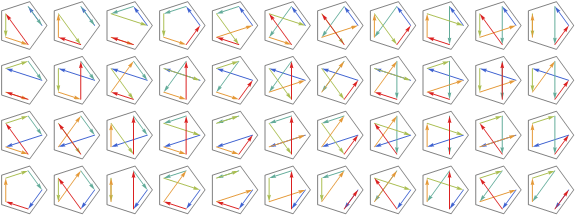# Derangements

A derangement (or complete permutation) of a set is a permutation that leaves no element in its original position. The number of derangements of a set with n elements can be computed recursively using this formula:

D(n + 1) = n (D(n) + D(n–1))

Using the principle of inclusion and exclusion, we also get:Here are some diagrams that represent the derangements of sets with n elements.

3 elements, 2 derangements:4 elements, 9 derangements:5 elements, 44 derangements:The counts are also known as subfactorials, with notation !n.

Derangement formulas from Fred S. Roberts, Applied Combinatorics, Prentice-Hall, 1984.

Designed and rendered on various weekends using Mathematica versions 3.0, 6.0, and 7.0.